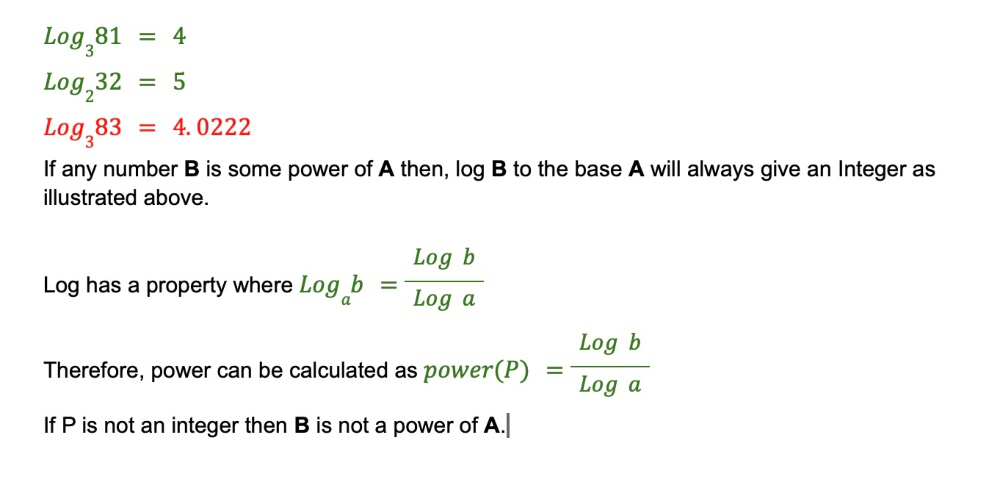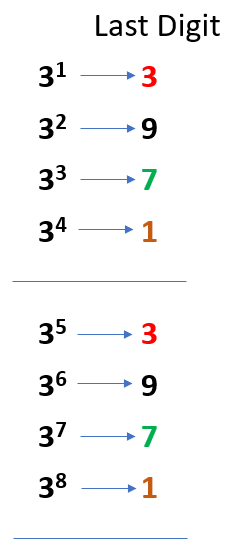# Find whether a given integer is a power of 3 or not

• Difficulty Level : Easy
• Last Updated : 18 Aug, 2022

Given a positive integer, write a function to find if it is a power of three or not.
Examples:

```Input : 3
Output :Yes

Input :6
Output :No```

General Solution to check if any number N is a power of P:Refer to the code below for implementation.

## C++

 `#include ``using` `namespace` `std;``#include ``int` `main()``{` `    ``int` `b = 81;``    ``int` `a = 3;``    ``// computing power``    ``double` `p = ``log10``(b) / ``log10``(a);``    ``// checking to see if power is an integer or not``    ``if` `(p - (``int``)p == 0) {``        ``cout<<``"YES"``;``    ``}``    ``else``{``        ``cout<<``"NO"``;``    ``}``    ``return` `0;``}` `// This code is contributed by ksrikanth0498.`

## Java

 `import` `java.io.*;` `class` `GFG {``    ``public` `static` `void` `main(String[] args)``    ``{``        ``int` `b = ``81``;``        ``int` `a = ``3``;``          ``// computing power``        ``double` `p = Math.log10(b)/ Math.log10(a);``        ``// checking to see if power is an integer or not``        ``if` `(p - (``int``)p == ``0``) {``            ``System.out.println(``"YES"``);``        ``}``        ``else``            ``System.out.println(``"NO"``);``    ``}``}` `/* This code is contributed by Ankit Agrawal */`

## C#

 `using` `System;` `public` `class` `GFG{` `     ``public` `static` `void` `Main()``    ``{``        ``int` `b = 81;``        ``int` `a = 3;``       ` `          ``// computing power``        ``double` `p = Math.Log10(b)/ Math.Log10(a);``       ` `        ``// checking to see if power is an integer or not``        ``if` `(p - (``int``)p == 0) {``            ``Console.Write(``"YES"``);``        ``}``        ``else``            ``Console.Write(``"NO"``);``    ``}``}` `// This code is contributed by laxmigangajula03.`

## Javascript

 ``

Output

`YES`

Time Complexity: O(1)

Space Complexity: O(1)

Recursive approach :

Check if the number is divisible by 3, if yes then keep checking the same for number/3 recursively. If the number can be reduced to 1, then the number is divisible by 3 else not.

## C++

 `#include ``#define ll long long``using` `namespace` `std;``bool` `isPower_of_Three(ll n)``{``    ``if` `(n <= 0)``        ``return` `false``;``    ``if` `(n % 3 == 0)``        ``return` `isPower_of_Three(n / 3);``    ``if` `(n == 1)``        ``return` `true``;``    ``return` `false``;``}``int` `main()``{``    ``ll num1;``    ``num1 = 243;``    ``if` `(isPower_of_Three(num1))``        ``cout << ``"Yes"` `<< endl;``    ``else``        ``cout << ``"No"` `<< endl;``    ``ll num2 = 6;``    ``if` `(isPower_of_Three(num2))``        ``cout << ``"Yes"` `<< endl;``    ``else``        ``cout << ``"No"` `<< endl;``    ``return` `0;``}` `// This code is contributed by Sania Kumari Gupta (kriSania804)`

## C

 `#include ``#include ` `#define ll long long` `bool` `isPower_of_Three(ll n)``{``    ``if` `(n <= 0)``        ``return` `false``;``    ``if` `(n % 3 == 0)``        ``return` `isPower_of_Three(n / 3);``    ``if` `(n == 1)``        ``return` `true``;``    ``return` `false``;``}``int` `main()``{``    ``ll num1;``    ``num1 = 243;``    ``if` `(isPower_of_Three(num1))``        ``printf``(``"Yes\n"``);``    ``else``        ``printf``(``"No\n"``);``    ``ll num2 = 6;``    ``if` `(isPower_of_Three(num2))``        ``printf``(``"Yes\n"``);``    ``else``        ``printf``(``"No\n"``);``    ``return` `0;``}` `// This code is contributed by Sania Kumari Gupta (kriSania804)`

## Java

 `import` `java.util.*;` `class` `GFG{``static` `boolean` `isPower_of_Three(``long` `n)``{``    ``if` `(n <= ``0``)``        ``return` `false``;``    ``if` `(n % ``3` `== ``0``)``        ``return` `isPower_of_Three(n / ``3``);``    ``if` `(n == ``1``)``        ``return` `true``;``    ``return` `false``;``}``  ` `  ``// Driver code``public` `static` `void` `main(String[] args)``{``    ``long`  `num1 = ``243``;``    ``if` `(isPower_of_Three(num1))``        ``System.out.print(``"Yes"` `+``"\n"``);``    ``else``        ``System.out.print(``"No"` `+``"\n"``);``    ``long` `num2 = ``6``;``    ``if` `(isPower_of_Three(num2))``        ``System.out.print(``"Yes"` `+``"\n"``);``    ``else``        ``System.out.print(``"No"` `+``"\n"``);``}``}` `// This code is contributed by umadevi9616`

## Python3

 `def` `isPower_of_Three(n):` `    ``if` `(n <``=` `0``):``        ``return` `False``    ``if` `(n ``%` `3` `=``=` `0``):``        ``return` `isPower_of_Three(n ``/``/` `3``)``    ``if` `(n ``=``=` `1``):``        ``return` `True``    ``return` `False` ` ``# Driver code``num1 ``=` `243``if` `(isPower_of_Three(num1)):``    ``print``(``"Yes"``)``else``:``    ``print``(``"No"``)``    ` `num2 ``=` `6``if` `(isPower_of_Three(num2)):``    ``print``(``"Yes"``)``else``:``    ``print``(``"No"``)` `# This code is contributed by shivanisinghss2110`

## C#

 `using` `System;` `class` `GFG{``static` `Boolean isPower_of_Three(``long` `n)``{``    ``if` `(n <= 0)``        ``return` `false``;``    ``if` `(n % 3 == 0)``        ``return` `isPower_of_Three(n / 3);``    ``if` `(n == 1)``        ``return` `true``;``    ``return` `false``;``}``  ` `  ``// Driver code``public` `static` `void` `Main(String[] args)``{``    ``long`  `num1 = 243;``    ``if` `(isPower_of_Three(num1))``        ``Console.Write(``"Yes"` `+``"\n"``);``    ``else``        ``Console.Write(``"No"` `+``"\n"``);``    ``long` `num2 = 6;``    ``if` `(isPower_of_Three(num2))``        ``Console.Write(``"Yes"` `+``"\n"``);``    ``else``        ``Console.Write(``"No"` `+``"\n"``);``}``}` `// this code is contributed by shivanisinghss2110`

## Javascript

 ``

## PHP

 ``

Output

```Yes
No```

Time Complexity: O(log3n), where n represents the given integer.
Auxiliary Space: O(log3n).

Approach:
The logic is very simple. Any integer number other than power of 3 which divides highest power of 3 value that integer can hold 3^19 = 1162261467 (Assuming that integers are stored using 32 bits) will give reminder non-zero.

## C++

 `// C++ program to check if a number is power``// of 3 or not.``#include ``using` `namespace` `std;` `// Returns true if n is power of 3, else false``bool` `check(``int` `n)``{``    ``if` `(n <= 0)``        ``return` `false``;``  ` `    ``/* The maximum power of 3 value that``       ``integer can hold is 1162261467 ( 3^19 ) .*/``    ``return` `1162261467 % n == 0;``}` `// Driver code``int` `main()``{``    ``int` `n = 9;``    ``if` `(check(n))``        ``cout <<``"Yes"``;``    ``else``        ``cout <<``"No"``;` `    ``return` `0;``}` `// This code is contributed by shivanisinghss2110`

## C

 `// C++ program to check if a number is power``// of 3 or not.``#include ``#include ` `// Returns true if n is power of 3, else false``bool` `check(``int` `n)``{``    ``if` `(n <= 0)``        ``return` `false``;``    ``/* The maximum power of 3 value that``       ``integer can hold is 1162261467 ( 3^19 ) .*/``    ``return` `1162261467 % n == 0;``}` `// Driver code``int` `main()``{``    ``int` `n = 9;``    ``if` `(check(n))``        ``printf``(``"Yes"``);``    ``else``        ``printf``(``"No"``);` `    ``return` `0;``}`

## Java

 `// Java program to check if a number is power``// of 3 or not.``public` `class` `Power_3 {` `    ``// Returns true if n is power of 3, else false``    ``static` `boolean` `check(``int` `n)``    ``{``        ``/* To prevent``        ``java.lang.ArithmeticException: / by zero and``        ``negative n */``        ``if` `(n <= ``0``)``            ``return` `false``;``        ``/* The maximum power of 3 value that``           ``integer can hold is 1162261467 ( 3^19 ) .*/``        ``return` `1162261467` `% n == ``0``;``    ``}` `    ``// Driver code``    ``public` `static` `void` `main(String args[])``    ``{``        ``int` `n = ``9``;``        ``if` `(check(n))``            ``System.out.println(``"Yes"``);``        ``else``            ``System.out.println(``"No"``);``    ``}``}``// This code is contributed by Sumit Ghosh`

## Python

 `# Python program to check if a number is power``# of 3 or not.`` ` `# Returns true if n is power of 3, else false``def` `check(n):``    ``""" The maximum power of 3 value that``       ``integer can hold is 1162261467 ( 3^19 ) ."""``    ``return` `1162261467` `%` `n ``=``=` `0`` ` `# Driver code``n ``=` `9``if` `(check(n)):``    ``print` `(``"Yes"``)``else``:``    ``print` `(``"No"``)` `# This code is contributed by Sachin Bisht`

## C#

 `// C# program to check if a number``// is power of 3 or not.``using` `System;` `public` `class` `GFG {` `    ``// Returns true if n is power``    ``// of 3, else false``    ``static` `bool` `check(``int` `n)``    ``{``        ``if` `(n <= 0)``            ``return` `false``;``        ``/* The maximum power of 3``        ``value that integer can hold``        ``is 1162261467 ( 3^19 ) .*/``        ``return` `1162261467 % n == 0;``    ``}` `    ``// Driver code``    ``public` `static` `void` `Main()``    ``{``        ``int` `n = 9;``        ``if` `(check(n))``            ``Console.Write(``"Yes"``);``        ``else``            ``Console.Write(``"No"``);``    ``}``}` `// This code is contributed by``// nitin mittal.`

## PHP

 ``

## Javascript

 ``

Output

`Yes`

Time Complexity : O(1)

Auxiliary Space: O(1)

Approach:

This approach is based on the below simple observations.Observation 1: If there is a power of three number, it will definitely end with either 3, 9 , 7 or 1.

Observation 2 : If a number ends with one of these 4 digits, we only have to check the powers of three which would guarantee a number ending with that last digit. For example, if a given number ends with 1, it must be a 4th or 8th or 12th and so on power of three, if at all.

Now since we are clear with the observations, let’s have a look at the algorithm.

Algorithm :

Step 1: If the given number, n, is not ending with 3,9,7 or 1, it means that the number is not a power of three, therefore return FALSE.

Step 2 : If not, we create a Map with 4 entries in it in order to maintain the mapping between the powers to three(1,2,3,4) and the number’s last digits(3,9,7,1).

Step 3 : Extract the last digit from a given number and look up it’s corresponding power in the map.

Step 4 :  If this power when raised to three equals  the number, n, return TRUE.

Step 5 : If this power raised to three is less than the number, n, increment the power straight by 4 and loop step 4 until the power raised to three becomes more than n.

Step 6 : If the power raised to three becomes more than the given number, return FALSE.

## C++

 `#include ``using` `namespace` `std;``bool` `isPowerOfThree(``int` `n)``{``    ``if` `(n == 1)``        ``return` `true``;``    ``int` `lastDigit = n % 10;``    ``map<``int``, ``int``> map;``    ``map = 1;``    ``map = 2;``    ``map = 3;``    ``map = 4;` `    ``if` `(!map[lastDigit])``        ``return` `false``;` `    ``int` `power = map[lastDigit];``    ``double` `powerOfThree = ``pow``(3, power);``    ``while` `(powerOfThree <= n) {``        ``if` `(powerOfThree == n)``            ``return` `true``;``        ``power = power + 4;``        ``powerOfThree = ``pow``(3, power);``    ``}``    ``return` `false``;``}``int` `main()``{``    ``int` `n = 81;``    ``cout << (isPowerOfThree(n) ? ``"true"` `: ``"false"``) << endl;``    ``n = 91;``    ``cout << (isPowerOfThree(n) ? ``"true"` `: ``"false"``) << endl;``    ``return` `0;``}` `// This code is contributed by umadevi9616`

## Java

 `/*package whatever //do not write package name here */` `import` `java.io.*;``import` `java.util.*;` `class` `GFG {``    ``public` `static` `boolean` `isPowerOfThree(``int` `n)``    ``{``        ``if` `(n == ``1``)``            ``return` `true``;``        ``int` `lastDigit = n % ``10``;``        ``Map map = ``new` `HashMap<>();``        ``map.put(``3``, ``1``);``        ``map.put(``9``, ``2``);``        ``map.put(``7``, ``3``);``        ``map.put(``1``, ``4``);` `        ``if` `(map.get(lastDigit) == ``null``)``            ``return` `false``;` `        ``int` `power = map.get(lastDigit);``        ``double` `powerOfThree = Math.pow(``3``, power);``        ``while` `(powerOfThree <= n) {``            ``if` `(powerOfThree == n)``                ``return` `true``;``            ``power = power + ``4``;``            ``powerOfThree = Math.pow(``3``, power);``        ``}``        ``return` `false``;``    ``}``    ``public` `static` `void` `main(String[] args)``    ``{``        ``int` `n = ``81``;``        ``System.out.println(isPowerOfThree(n));``        ``n = ``91``;``        ``System.out.println(isPowerOfThree(n));``    ``}``}`

## Python3

 `'''package whatever #do not write package name here '''``def` `isPowerOfThree(n):``    ``if` `(n ``=``=` `1``):``        ``return` `True``;``    ``lastDigit ``=` `n ``%` `10``;``    ``map` `=``[``0``] ``*` `1000``;``    ``map``[``3``] ``=` `1``;``    ``map``[``9``] ``=` `2``;``    ``map``[``7``] ``=` `3``;``    ``map``[``1``] ``=` `4``;` `    ``if` `(``map``[lastDigit] ``=``=` `None``):``        ``return` `False``;` `    ``power ``=` `map``[lastDigit];``    ``powerOfThree ``=` `pow``(``3``, power);``    ``while` `(powerOfThree <``=` `n):``        ``if` `(powerOfThree ``=``=` `n):``            ``return` `True``;``        ``power ``=` `power ``+` `4``;``        ``powerOfThree ``=` `pow``(``3``, power);``    ` `    ``return` `False``;` `if` `__name__ ``=``=` `'__main__'``:``    ``n ``=` `81``;``    ``print``(isPowerOfThree(n));``    ``n ``=` `91``;``    ``print``(isPowerOfThree(n));` `# This code contributed by umadevi9616`

## C#

 `/*package whatever //do not write package name here */``using` `System;``using` `System.Collections.Generic;` `public` `class` `GFG {``    ``public` `static` `bool` `isPowerOfThree(``int` `n)``    ``{``        ``if` `(n == 1)``            ``return` `true``;``        ``int` `lastDigit = n % 10;``        ``Dictionary<``int``, ``int``> map = ``new` `Dictionary<``int``,``int``>();``        ``map.Add(3, 1);``        ``map.Add(9, 2);``        ``map.Add(7, 3);``        ``map.Add(1, 4);` `        ``if` `(!map.ContainsValue(lastDigit))``            ``return` `false``;` `        ``int` `power = map[lastDigit];``        ``double` `powerOfThree = Math.Pow(3, power);``        ``while` `(powerOfThree <= n) {``            ``if` `(powerOfThree == n)``                ``return` `true``;``            ``power = power + 4;``            ``powerOfThree = Math.Pow(3, power);``        ``}``        ``return` `false``;``    ``}``    ``public` `static` `void` `Main(String[] args)``    ``{``        ``int` `n = 81;``        ``Console.WriteLine(isPowerOfThree(n));``        ``n = 91;``        ``Console.WriteLine(isPowerOfThree(n));``    ``}``}` `// This code is contributed by umadevi9616`

## Javascript

 ``

Output

```true
false```

Analysis:

Runtime Complexity:

O(1) : Since the given number is an Integer, it can at max be 2147483647 (32 bit) and the highest power of three that is less than or equal to this number is 3^19 = 1162261467. And since we increment the power by 4, we will have a loop running at most 5 times, hence O(1).

Space Complexity:

O(1) : Since we only have 4 entries in a Map no matter how big the number is given to us.

This approach is contributed by Durgesh Valecha.

If you like GeeksforGeeks and would like to contribute, you can also write an article using write.geeksforgeeks.org or mail your article to review-team@geeksforgeeks.org. See your article appearing on the GeeksforGeeks main page and help other Geeks.